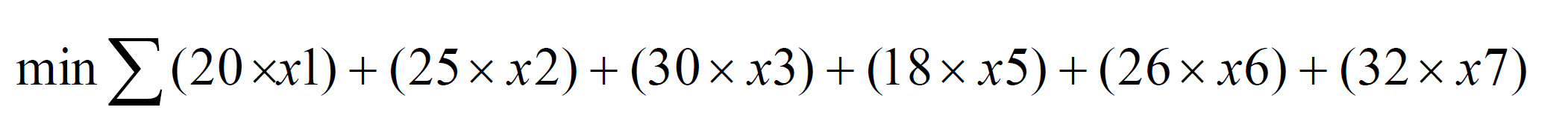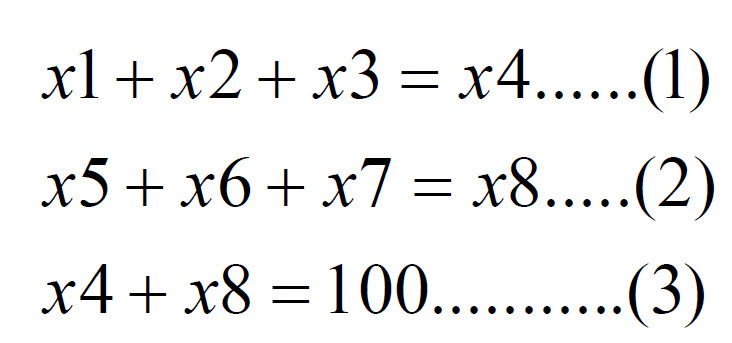# Linear Programming and Generator Economics

## Using Linear Programming to Minimize Generator Bid Costs

Linear Programming is used in power market economics and operations to minimize the cost of generator providers while considering constraints such as minimum up-time, bands, min/max mw, capability curves, etc.

My simple project example considers the market economics of two generators using linear programming to minimize costs with respect to constraints.

### Variables

There are 8 variables in my generator model problem: x1, x2, x3, x4, x5, x6, x7,x8. They are defined as given below:

 Variable Definition x1 G1 Bidding Quantity 20 MW Step x2 G1 Bidding Quantity 30 MW Step x3 G1 Bidding Quantity 15 MW Step x4 G1 = x1 + x2 + x3 or total MW to be bid by G1 x5 G2 Bidding Quantity 18 MW Step x6 G2 Bidding Quantity 26 MW Step x7 G2 Bidding Quantity 32 MW Step x8 G2 = x5 + x6 + x7 or total MW to be bid by G2

### Objective Function:

The objective of the problem is to minimize cost of combined bids of both generators.mathematical objective function

The objective function is the sum of the unit price of the MW multiplied by the quantity to be bid added to the other bid ranges and their respective products.

Note: In the MATLAB portion of the model, the x4 and x8 variables are set equal to 0 since it is not in the problem definition to minimize these.

### Constraints

The constraints of the problem are shown below:mathematical constraints

Constraint (1) is formulated on the definition that the Generator G1 bids from the given three quantities 20MW, 30MW, or 15 MW. Constraint (2) is formulated on the definition that Generator G2 bids from the given three quantities 15MW, 40MW, or 25MW.

### Bounds

The bounds of the model are defined in the problem statement and are shown below:

 Variable Lower Bound Upper Bound x1 0 20 x2 0 30 x3 0 15 x4 15 65 x5 0 15 x6 0 40 x7 0 25 x8 10 80

## Optimal Solution

### Accepted Quantity

The accepted quantity from each generator range is shown below and in the MATLAB results (attached code):

G1 Bidding Information

 Quantity (MW) Price (\$/MWH) 20 20 30 25 0 30

G2 Bidding Information

 Quantity (MW) Price (\$/MWH) 15 18 35 26 0 32

The minimized cost under the optimal model  to supply 100 MW load is \$2,330.00. The cost to supply load by generator G1 will be \$1150 from G2, \$1180.

## My Solution Code